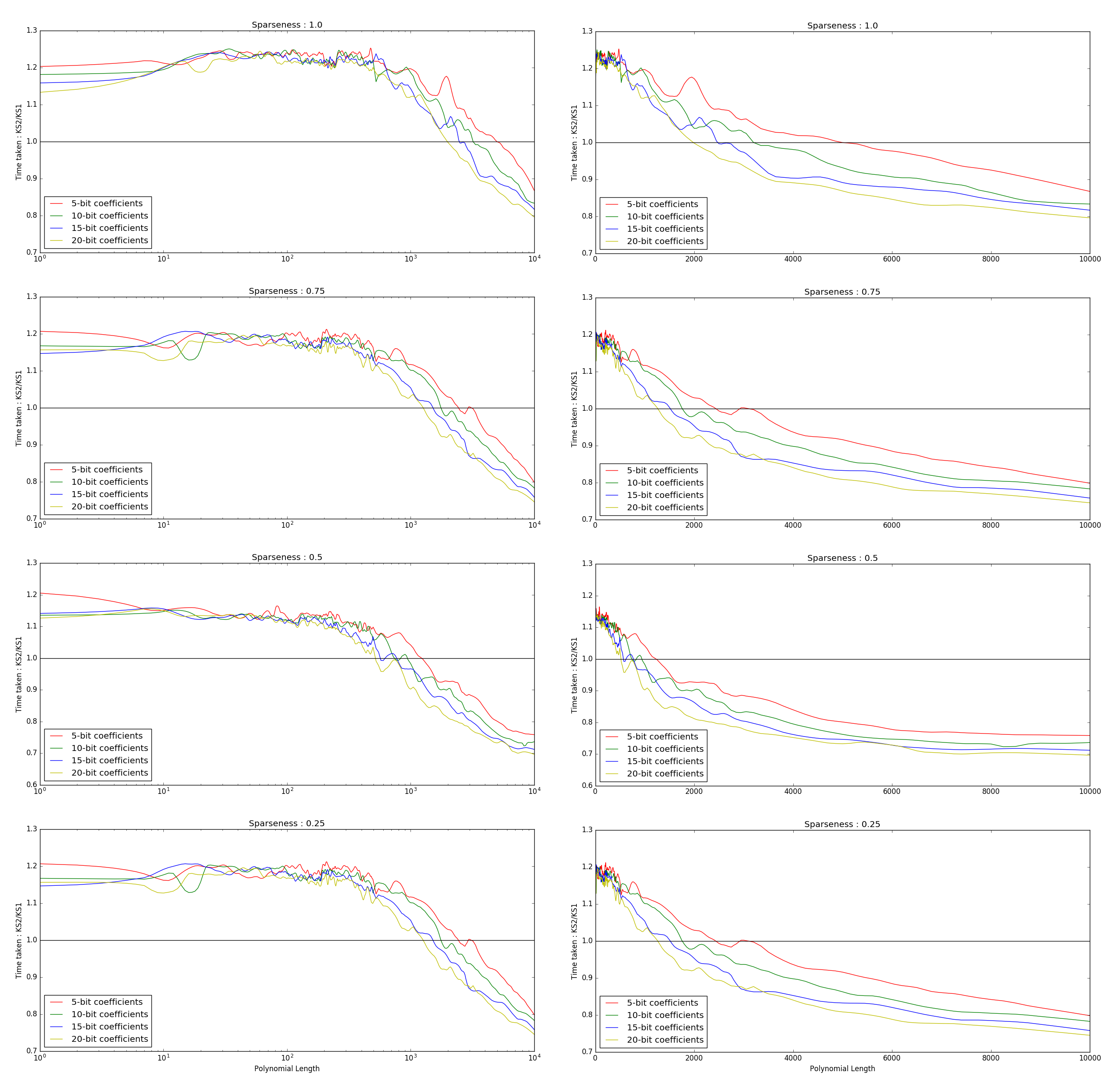# Pre GSoC and Community Bonding

### The Kronecker Substitution

I started off my work by reading through the existing `mul_poly` function. It uses the Kronecker Substitution technique to multiply two polynomials. An insight can be gained by looking at the slides here. Think of it this way,

“If you evaluate a polynomial at a large enough power of 10, I bet you can tell all it’s coefficients just by looking at the result!”

The mentioned slides call this the KS1 algorithm. Another algorithm it proposes is the KS2 algorithm, which evaluates the polynomial at two points (in contrast to just one) to interpolate the polynomial. A more mathematical explanation on the two techniques can be found here. I implemented the algorithm, and it wasn’t too difficult, as it was a a slight modification to the already existing multiplication technique. Later, I added a benchmark test for comparing the two techniques, KS1 & KS2. The benchmark (roughly) calculates the ratio of the time required for multiplying two polynomials using the two algorithms. Both the polynomial length (from 1 to 10,000) and the bit length of the coefficients (5, 10, 15, 20 bits) were varied. The graphs of the benchmarking are as follows.

Linear & Log scale :During this time, I was asked by Isuru to switch work towards the polynomial interface with FLINT & Piranha (and shift the polynomial manipulations to the end of summer). So, the PR hasn’t been merged in yet, and no conclusions and observations have been made between the two algorithms as of yet. Will be done later during the summer. Here’s the PR #930

### Dictionary wrappers

I also started work on Dictionary wrappers for SymEngine. One was already made, for the `UnivariatePolynomial` class aka the class for univariate polynomials with symbolic coefficients. It is a map from `int -> Expression`. We needed another wrapper for the `uint -> integer_class` map, so that the `UnivariateIntPolynomial` class can be structured the same way as the former. Now that we need almost the same functionality, why not temlatize the wrapper? (suggested by Isuru) That’s what I did, and the PR #946 is almost merged in. More on wrappers next time!

### Miscellaneous issues

Most of my work during this period revolved around reading the existing polynomial class, and refactor it and removed any redundancies. Some of the miscellaneous work that was done :

• Some refactoring was done in the `dict.cpp` file. There were some redundancy in the functions which was removed. Templatized methods for checking equality and comparing vectors (and sets) were made. Other specific `eq` & `compare` methods became derived methods of these base classes. #933

• Initially, the `mul_poly` method was constructing a vector of coefficients for the resulting multiplied polynomial (thus, implicitly storing it in a dense representation for a while). However, it was returned as a sparse represented polynomial, using a dictionary. This was changed, so that the dictionary is directly created, and the intermediate vector isn’t requireds. Also, some changes in variable names for clarity, as well as removing the redundant function `dict_add_term`. #928

• A redundant function `create` was removed. All it was doing was calling `from_vec` within the same class. #941

See you next week, Goodbye!